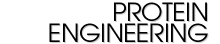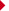Similar articles found in:Protein Eng. OnlinePubMedPubMed CitationThis Article has been cited by:other online articlesSearch Medline for articles by:Miyazawa, S.Alert me when:new articles cite this articleDownload to Citation Manager

Protein Engineering, Vol 8, 999-1009, Copyright Ź˘í 1995 by Oxford University Press

## A reliable sequence alignment method based on probabilities of residue correspondences

S Miyazawa
Faculty of Technology, Gunma University, Japan.

Probabilities of all possible correspondences of residues in aligning two proteins are evaluated by assuming that the statistical weight of each alignment is proportional to the exponent of its total similarity score. Based on such probabilities, a probability alignment that includes the most probable correspondences is proposed. In the case of highly similar sequence pairs, the probability alignments agree with the maximum similarity alignments that correspond to the alignments with the maximum similarity score. Significant correspondences in the probability alignments are those whose probabilities are > 0.5. The probability alignment method is applied to a few protein pairs, and results indicate that such highly probable correspondences in the probability alignments are probably correct correspondences that agree with the structural alignments and that incorrect correspondences in the maximum similarity alignments are usually insignificant correspondences in the probability alignments. The root mean square deviations in superimposition of corresponding residues tend to be smaller for significant correspondences in the probability alignments than for all correspondences in the maximum similarity alignments, indicating that incorrect correspondences in the maximum similarity alignments tend to be insignificant correspondences in probability alignments. This fact is also confirmed in 109 protein pairs that are similar to each other with sequence identities between 90 and 35%. In addition, the probability alignment method may better predict correct correspondences than the maximum similarity alignment method. Probability alignments do, of course, depend on a scoring scheme but are less sensitive to the value of parameters such as gap penalties. The present probability alignment method is useful for constructing reliable alignments based on the probabilities of correspondences and can be used with any scoring scheme.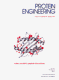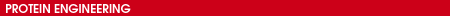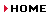S. Miyazawa and R. L. Jernigan Identifying sequence-structure pairs undetected by sequence alignments Protein Eng., July 1, 2000; 13(7): 459 - 475. [Abstract] [Full Text] [PDF]